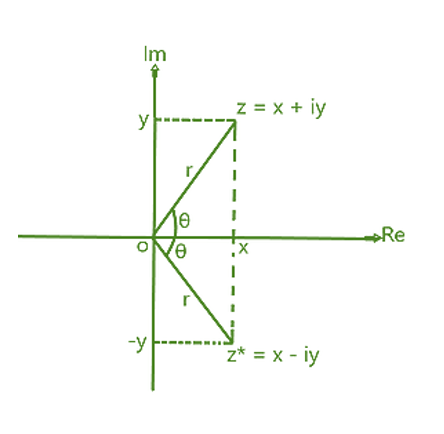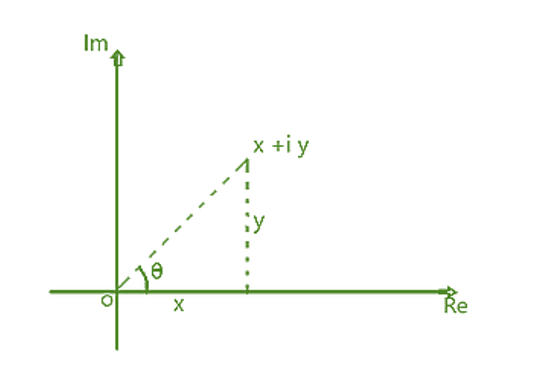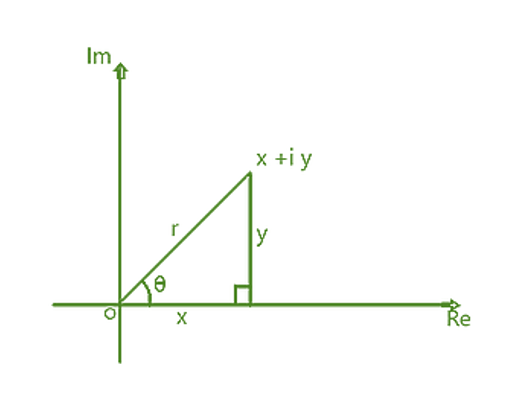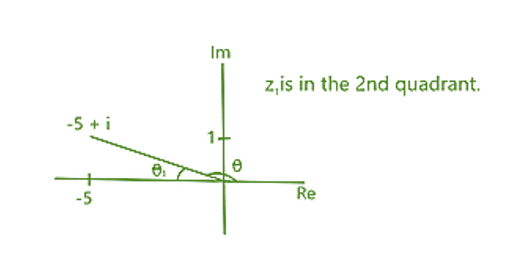GeeksforGeeks App
Open AppBrowser
Continue

# Complex Number Formula

The sum of a real number and an imaginary number is defined as a complex number, and the numbers which are not real numbers are called imaginary numbers. The number can be written in form of b+ic, where b and c are real numbers and ic is an imaginary number, and ” i” is an imaginary part which is called iota. hence here the value of i is (√-1) . so i2=-1

Let’s think about the equation x2+16=0

You could rewrite it as x²=-16. However, since the square of every real number is positive or zero, there is no real number with a square of -16. This is an example of a quadratic equation that, up to now, you would have classified as having ‘no real roots”.

The existence of such equations was recognized for hundreds of years, in the same way, that Greek mathematicians had accepted that x2 + 16 = 0 had no solution, the concept of a negative number had yet to be developed. The number system has expanded as mathematicians increased the range of mathematical problems they wanted to tackle.

You can solve the equation  x2+16=0 by extending the number system to include a new number, i (sometimes written as j). This has the property that i2 = -1 and it follows the usual laws of algebra, i is called an imaginary number.

The square root of any negative number can be expressed in terms of i. For example, the solution of the equation  x2 = -16 is x = ±√-16. This can be written as

±√16 * √-1 which simplifies to ±4i.

Example: Use the quadratic formula to solve the quadratic equation z2 – 6z + 58 = 0, simplifying your answer as far as possible.

Solution:

z2 – 6z + 58 = 0                     (Using the Quadratic formula with a = 1,b = -6 and c = 58)

z = (6 ±√((-6)2 – 4*1*58)) / (2*1)

z = (6 ±√(-196)) / 2                (as √-196  = √196 * √-1 = 14i) (also √196 = 14)

z = (6 ± 14i) / 2

z = 3 ± 7i

You will have noticed that the roots 3 + 7i and 3 – 7i of the quadratic equation z2 – 6z + 58 = 0 have both a real part and an imaginary part.

If we consider the complex number z = 3 + 7i then 3 is called the real part of z i.e Re(z) and 7 is called the imaginary part of z i.e Im(z).

### Notation

Any number z of the form x + yi, where x and y are real, is called a complex number. The letter z is commonly used for complex numbers, and w is also used. In this

chapter, a complex number z is often denoted by x + yi, but other letters are sometimes used, such as a + bi.

x is called the real part of the complex number, denoted by Re(z) and y is called the imaginary part, denoted by Im(z).

### Working with complex numbers

The general methods for addition, subtraction, and multiplication of complex numbers are straightforward.

Add the real part with real part and add the imaginary part with imaginary part.

For example, (3 + 4i) + (2 – 8i) = (3 + 2) + (4 – 8)i

= 5 – 4i

Subtraction

Subtract the real part with real part and subtract the imaginary part with imaginary part.

For example, (6 – 9i) – (1 + 6i) = 5 – 15i

Multiplication

Multiply out the complex numbers using distributive property of multiplication.

For example

(7 + 2i) (3 – 4i) = 21 – 28i + 6i – 8i2

= 21 – 22i – 8(-1)                        ( as i2 = -1)

= 29 – 22i

### Complex conjugates

The complex conjugate of a complex number is another complex number whose real part is the same as the original complex number and whose imaginary part has the same magnitude with opposite signs. Complex numbers are of the form a + ib. Where a and b are real numbers, a is called the real part of a+ib, and ib is called the imaginary part of a+ib.

The complex conjugate of a + ib with the real part “a” and the imaginary part “ib” is given by a – ib with the real part “a” and the imaginary part “-ib“. a – ib is a reflection of a + ib centered on the real axis (X-axis) of the Argand plane. Complex conjugates of complex numbers are used to rationalize complex numbers.

The product of a complex number and its complex conjugate is equal to the square of the magnitude of the complex number which gives a real number i.e.

z.z*= |z|2 = √(x2+y2)Example: Find the complex conjugate of the complex number 3z + iw, if z = 1 – i and w = 2 – i.

Solution:

First of all we will simplify 3z + iw = 3(1 – i) + i( 2 – i) = 3 – 3i + 2i + 1 = 4 – i

To determine the complex conjugate of 3z + iw = 4 – i, we will change the sign of i.

Therefore the complex conjugate of 4 – i is 4 + i.

### Equality of complex numbers

Two complex numbers z = x + yi and w = u + vi are equal if both x = u and y = v.

If x ≠ u or y ≠ v, or both, then z and w are not equal. You may feel that this is obvious, but it is interesting to compare this situation with the equality of rational numbers.

For two complex numbers to be equal the real parts must be equal and the imaginary parts must be equal. Using this result is described as equating real and imaginary parts, as shown in the following example.

Example: The complex numbers z1 and z2 are given by

z1 = (3 – a) + (2b – 4)i

z2 = (7b – 4) + (3a – 2)i.

Given that z1 and z2 are equal, find the values of a and b.

Solution:

Here, if we equate the real part of z1 and z2 then

3 – a = 7b – 4 ⇒ 7b + a = 7        (1)

and if we equate the imaginary part of z1 and z2 then

2b – 4 = 3a – 2 ⇒ 3a – 2b = -2    (2)

solving (1) and (2) by elimination method , we get

a =0 and b = 1

Example: Given that = 3 + 5i, find

(i) z +(Here= a – ib which is called as the Conjugate of z)

(ii) z×Solution:

z= 3 + 5i,

then \bar{z} = 3-5i

(i)   z += (3 + 5i) + (3 – 5i) = 6

(ii)  z×= (3 + 5i) * (3 – 5i) = 9 + 15i – 15i – 25 i2                  (i2 = -1)

=9 + 25

= 34

You can see from the example above that z +and zare both real. This is an example of an important general

Important Result:

That the sum of two complex conjugates is real and that their product is also real.

### Dividing complex numbers

You probably already know that you can write an expression like 2 / (3 – √2) as a fraction with a rational denominator by multiplying the numerator and denominator by 3 + √2.

2 / (3 – √2) = ( 2 / (3 – √2)) * (3 + √2)/(3 + √2)

= (6 + 2√2) / (9 – 2)

= (6 + 2√2) / 7

Because zis always real, you can use a similar method to write an expression like 2 / (3 – 5i) as a fraction with a real denominator, by multiplying the numerator

and denominator by 3+ 5i.                  (as 3 + 5i is the complex conjugate of 3 – 5i)

This is the basis for dividing one complex number by another.

Example: Find the real and imaginary parts of 1/(3 + i).

Solution

Multiply the numerator and denominator by 3 – i

(As  3 – i is the conjugate of the denominator  3 + i)

1/( 3 + i) = ( 3 – i) / [( 3 + i)( 3 – i]

= ( 3 – i) / (9 + 1)

= ( 3 – i) / 10

The real part is 3/10 and the imaginary part is -1/10.

### Modulus and Argument of complex numbers

The below figure shows the point representing z = x + iy on an argand diagram.The distance of this point from the origin is √x² + y².

This distance is called the modulus of z and is denoted by |z|.

So, for the complex number z = x + yi,

|z| = √x² + y² .

Notice that z\bar{z} =  (x + iy)(x – iy) = x² + y², then |z| = z×\bar{z}

The argument i.e angle θ is measured anticlockwise from the positive real axis. By convention, the argument is measured in radians.

Arg z (θ) = tan¯1(b/a).

### Modulus-argument form / Polar form of a complex number

In the below figure, you can see the relationship between the components of a complex number and its modulus and argument.Using trigonometry, you can see that sinθ = y/r and so y = rsinθ.

Similarly, cosθ = x/r so x = rcosθ.

Therefore, the complex number z = x + yi can be written

z = r cosθ + r sinθ i

or

z = r (cosθ + i sinθ)

This is called the modulus-argument form / Polar form of the complex number and is sometimes written as (r, θ).

### Sample Problems

Question 1: Solve the equation (2 + 3i)z = 9 – 4i.

Solution:

We have (2 + 3i)z = 9 – 4i

⇒ z = (9 – 4i) / (2 + 3i)

= [(9 – 4i) / (2 + 3i)] * [(2 – 3i)(2 – 3i)]

= (18 – 27i – 8i + 12i2) / (4 – 6i + 6i – 9i2)

= (6 – 35i) / 13

= (6 / 13) – (35 / 13)i

Question 2: Find the real and imaginary parts of 1/(5 + 2i).

Solution:

Multiply the numerator and denominator by 5 – 2i

(As 5 – 2i is the conjugate of the denominator 5 + 2i)

1/(5 + 2i) = (5 – 2i) / [(5 + 2i)(5 – 2i)]

= (5 – 2i) / (25 + 4)

= (5 – 2i) / 29

The real part is 5/29 and the imaginary part is -2/29.

Question 3: Write the following complex numbers in the modulus-argument form

(i) z1 = √3 + 3i

(ii) z2 = √3 – 3i

Solution:

(i) For z1 = √3 + 3i , we have

modulus |z1| = √(√3)2 + (3)2 = 2√3

θ = tan¯1(3 / √3 ) = π / 3

⇒ arg z1 = π / 3

so, z1 =  2√3(cos(π / 3) + i sin(π / 3))

(ii)  For z2 = √3 – 3i, we have

modulus |z2| = √(√3)2 + (3)2 = 2√3

θ = tan¯1(-3 / √3 ) = -π / 3

⇒ arg z2 = -π / 3

so, z2 =  2√3(cos(-π / 3) + i sin(-π / 3))

Question 4: For the given complex number, find the argument of the complex number, giving your answers in radians in exact form or to 3 significant

figures as appropriate.

(i) z1 = −5+ i

Solution:θ1 = tan¯1(1/5) = 0.1973…

so arg z1 = π – 0.1973… = 2.94 (3 s.f)

Question 5: Express the given complex number (-4) in the polar form.

Solution:

Given, complex number is -4 i.e z = -4 +0 i

Let r cos θ = -4 …(1)

and r sin θ = 0 …(2)

Now, squaring and adding (1) and (2), we get

r2cos2θ + r2sin2θ = (-4)2

r2(cos2θ + sin2θ) = 16

We know that, cos2θ + sin2θ = 1, then the above equation becomes,

r2 = 16

r = 4 (Conventionally, r > 0)

Now, substitute the value of r in (1) and (2), we get

4 cos θ = -4 and 4 sin θ = 0

⇒ cos θ = -1 and sin θ = 0

Therefore, θ = π

Hence, the polar representation is,

-4 = r cos θ + i r sin θ

4 cos π + 4i sin π = 4(cos π + i sin π)

Thus, the required polar form is 4 cos π+ 4i sin π = 4(cos π+i sin π).

My Personal Notes arrow_drop_up
Related Tutorials